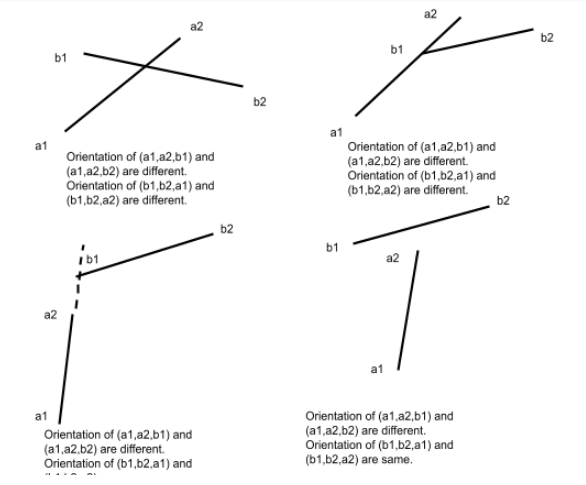# Check if two line segments intersect

## Introduction

The problem of checking if two line segments intersecting can be solved using the concept of coordinate geometry. Here we will use some formula that comes from coordinate geometry.

## Problem Statement

For two line segments (a1,a2) and (b1,b2), check if these two are intersecting where a1,a2 endpoints of the first line segment and b1,b2 endpoints of the second segment.

## Example

Input: a1=(1,5), a2=(5,8)  ; b1=(2,1); b2=(3,10)

Output:Yes (i.e intersecting)

## Prerequisite

Orientation of ordered triplet of points p1,p2, and p3 depends on the value of the below expression.

exp = (p2.y - p1.y)*(p3.x - p2.x) - (p2.x - p1.x)*(p3.y - p2.y)

Where p.x and p.y are respectively x and y coordinates of point p.

[ this relation can be  proved from the slope of lines]

If exp=0, the orientation is collinear; if exp>0, orientation is clockwise, and if exp<0, the orientation is counterclockwise.

Code

## Method

To know if two line segments are intersecting or not, we need to know about the orientation of their endpoints a1,a2,b1, and b2. Here we will consider the orientation of the ordered triplet of endpoints. Orientation of ordered triplet can be clockwise, counterclockwise or colinear.

In general  if (a1,a2,b1), (a1,a2,b2) these two ordered triplets have different orientation and (b1,b2,a1), (b1,b2,a2) these two ordered triplets have different orientation, two line segments will intersect.Otherwise, one endpoint of a line segment can lie on the other line segment. So

(a1,a2,b1) or (a1,a2,b2) or (b1,b2,a1) or (b1,b2,a2) can be collinear. In this case also two line segments are intersecting.

Output

## FAQs

### What is orientation?

Orientation of a triplet is how these points lie on a 2D plane. Orientation of ordered triplet can be clockwise, counterclockwise or colinear. It depends on their coordinates.

### What is a line segment?

A segment of a line connecting two given points is known as a line segment.

### What is slope a line segment and its formula?

For a line segment connecting two points (x1,y1) and (x2,y2),

Slope =(y2 - y1) / (x2 - x1)

## Key Takeaways

This article covered the method of checking if two line segments intersect.

Side by side, we should also learn how to check if a point lies in the interior of a polygon as this concept is used there.

Apart from this, you can practice a wide range of coding questions commonly asked in interviews in CodeStudio. Along with coding questions, we can also find the interview experience of scholars working in renowned product-based companies here

Happy learning!